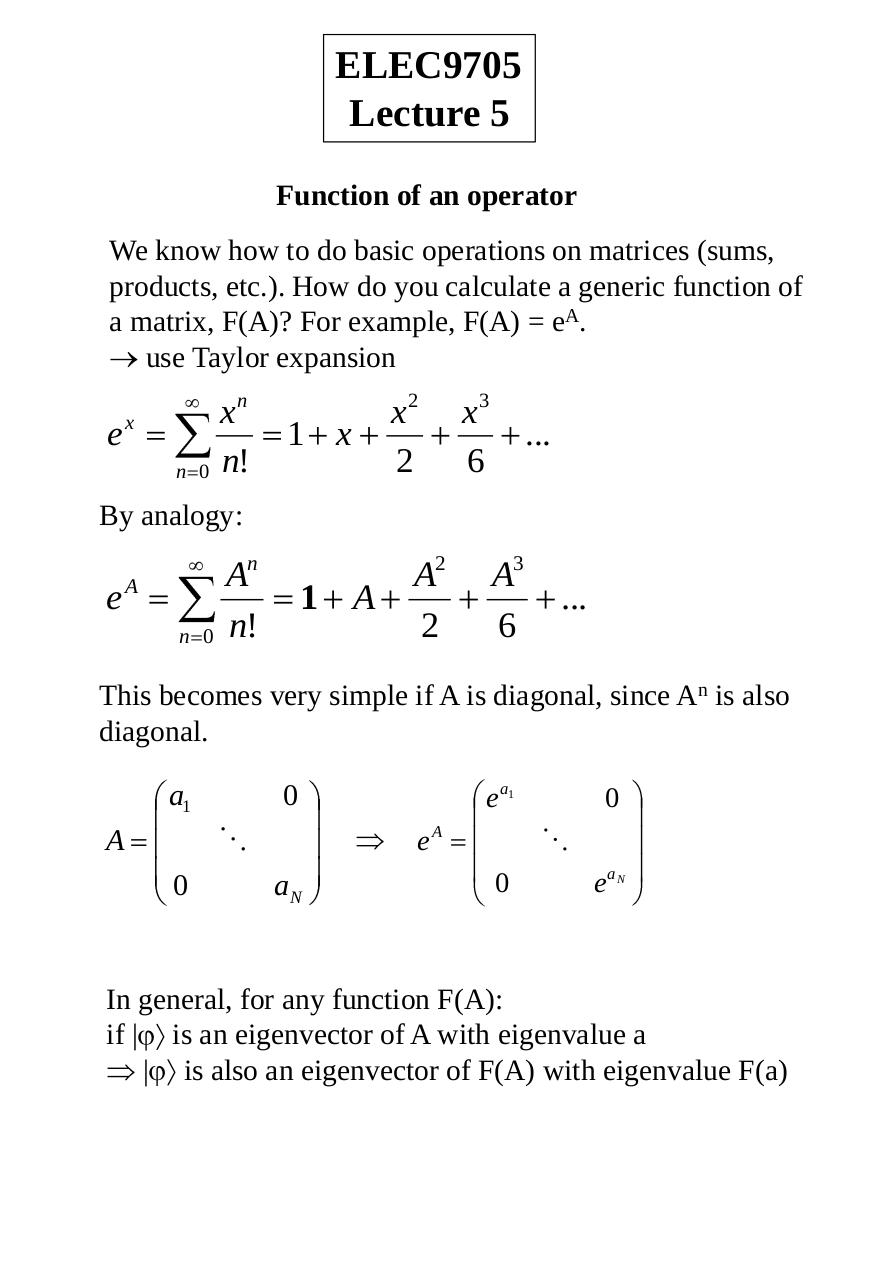# ELEC9705 lecture 05 Operators, Coupling, Entaglement.pdfPage 12315

#### Text preview

ELEC9705
Lecture 5
Function of an operator
We know how to do basic operations on matrices (sums,
products, etc.). How do you calculate a generic function of
a matrix, F(A)? For example, F(A) = eA.
use Taylor expansion

e

x
n 0

xn
n!

1 x

x2
2

x3
6

...

By analogy:

eA
n 0

An
n!

1 A

A2
2

A3
6

...

This becomes very simple if A is diagonal, since An is also
diagonal.

a1

eA

A
0

ea1

0
aN

0

0

eaN

In general, for any function F(A):
if | is an eigenvector of A with eigenvalue a
| is also an eigenvector of F(A) with eigenvalue F(a)